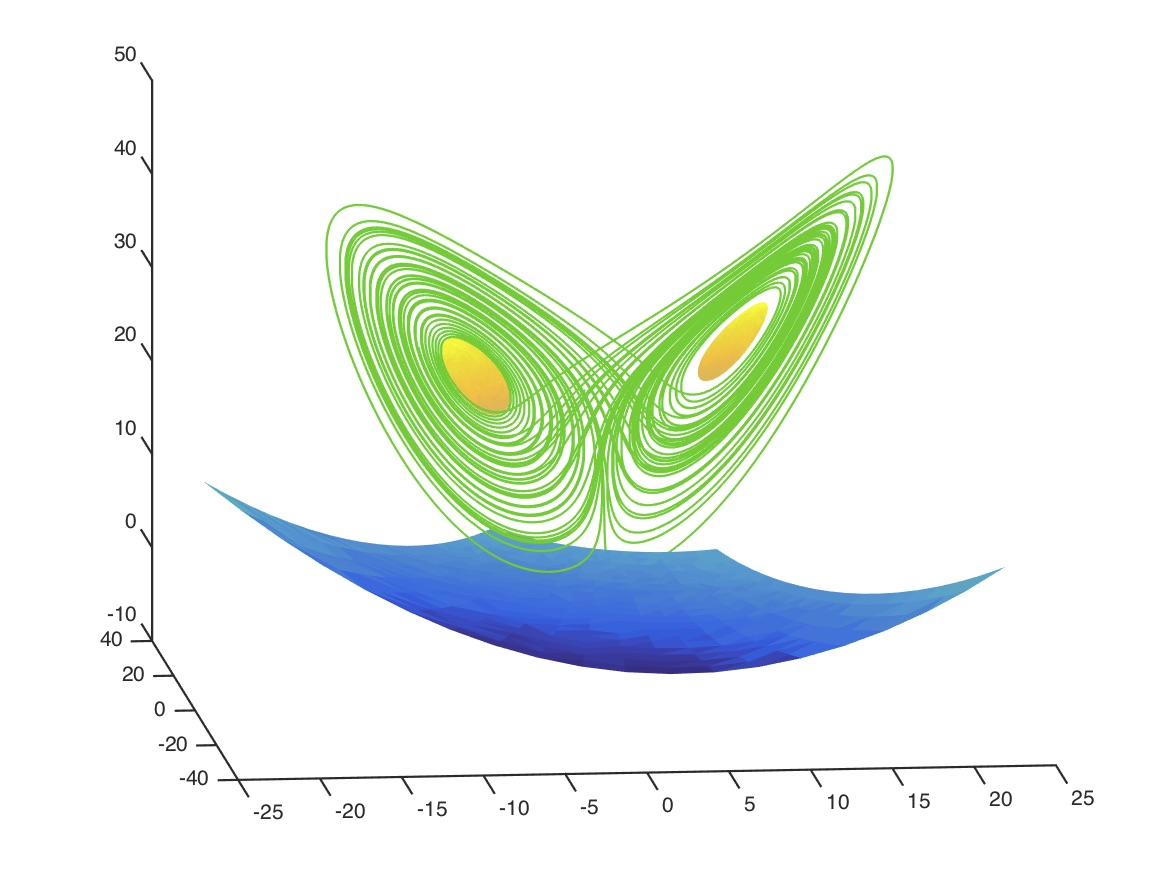### Computation of maximal local (un)stable manifold patches by the parameterization method

Maxime Breden, Jean-Philippe Lessard and Jason D. Mireles James

In this work we develop some automatic procedures for computing high order polynomial expansions of local (un)stable manifolds for equilibria of differential equations. Our method incorporates validated truncation error bounds, and maximizes the size of the image of the polynomial approximation relative to some specified constraints. More precisely we use that the manifold computations depend heavily on the scalings of the eigenvectors: indeed we study the precise effects of these scalings on the estimates which determine the validated error bounds. This relationship between the eigenvector scalings and the error estimates plays a central role in our automatic procedures. In order to illustrate the utility of these methods we present several applications, including visualization of invariant manifolds in the Lorenz and FitzHugh-Nagumo systems and an automatic continuation scheme for (un)stable manifolds in a suspension bridge problem.

The paper in its pdf form can be found here.

Here are the MATLAB codes.